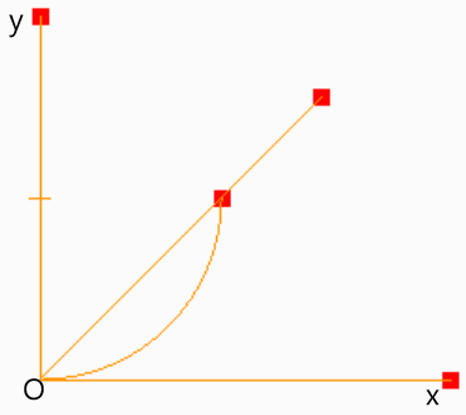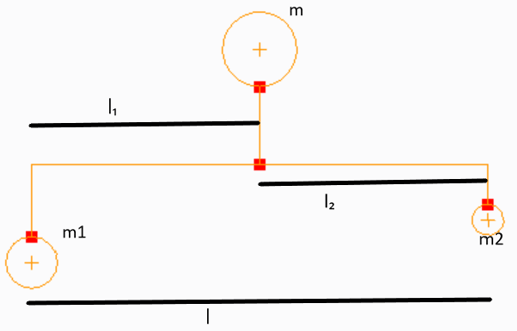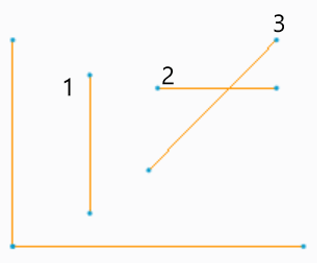Q.1
##### The graph plotted between the controlling force of the governor and Radius of rotation is known as _______
• a) Controlling force diagram
• b) Radius of rotation diagram
• c) Governor diagram
• d) Sensitivity diagram
Q.2
• a) True
• b) False
Q.3
##### In the given figure, the x – axis represents radius of rotation and the y axis represents the controlling force. This graph is known as _________• a) Controlling force diagram of Porter governor
• b) Controlling force diagram of Hartnell governor
• c) Controlling force diagram of isochronous governor
• d) Controlling force diagram of a general governor
Q.4
##### What should be the stability condition for the angle made by OA and the x axis? (the x – axis represents radius of rotation and the y axis represents the controlling force.)• a) Angle must increase
• b) Angle must decrease
• c) Angle must remain constant
• d) Stability is independent of this angle
Q.5
##### How should the equilibrium speed vary with the increase of radius of rotation of the governor balls for stability?
• a) Must increase
• b) Must decrease
• c) Must remain constant
• d) Equilibrium speed independent of radius of rotation
Q.6
##### For a more sensitive governor, the change in angle between OA and the x axis with change in radius of rotation should be? (the x – axis represents radius of rotation and the y axis represents the controlling force.)• a) Small
• b) Large
• c) Remain same
• d) Is independent of this angle
Q.7
##### In the given figure, the straight line OA represents _________ (the x – axis represents radius of rotation and the y axis represents the controlling force.)• a) Isochronous governor
• b) Hartnell governor
• c) Non – Isochronous governor
• d) Stable governor
Q.8
• a) True
• b) False